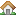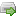•Home
•Purchase
•Downloads
•Manual
•Support Forum
You are here:  » Data not displaying

# Data not displaying

Submitted by baggagepin on Mon, 2010-05-17 15:04 in

Hi David,

Sorry to be a pain but can you see why the data is not displaying. The XML file is:

`<currencies><rate swift="AED" buy="6.2140" sell="5.0265"/><rate swift="AUD" buy="1.8637" sell="1.5387"/><rate swift="BBD" buy="3.3305" sell="2.6765"/><rate swift="BGN" buy="2.6410" sell="2.0753"/><rate swift="BRL" buy="3.1512" sell="2.3368"/><rate swift="CAD" buy="1.6938" sell="1.4049"/><rate swift="CHF" buy="1.8652" sell="1.5389"/><rate swift="CNY" buy="12.0086" sell="9.1803"/><rate swift="CZK" buy="35.1600" sell="26.1697"/><rate swift="DKK" buy="9.7593" sell="8.1427"/><rate swift="DOP" buy="62.5109" sell="46.9118"/><rate swift="EEK" buy="21.0356" sell="16.4491"/><rate swift="EGP" buy="9.4629" sell="7.3220"/><rate swift="EUR" buy="1.3240" sell="1.0950"/><rate swift="GMD" buy="45.8219" sell="34.3904"/><rate swift="HKD" buy="12.9380" sell="10.7947"/><rate swift="HRK" buy="9.8437" sell="7.7288"/><rate swift="HUF" buy="372.9939" sell="287.8186"/><rate swift="IDR" buy="17593.4300" sell="12073.4494"/><rate swift="ILS" buy="6.4379" sell="5.0972"/><rate swift="JMD" buy="154.8029" sell="118.5404"/><rate swift="JPY" buy="157.4042" sell="129.1855"/><rate swift="KES" buy="135.3369" sell="105.6276"/><rate swift="LTL" buy="4.5907" sell="3.5391"/><rate swift="LVL" buy="0.9366" sell="0.7533"/><rate swift="MUR" buy="52.7730" sell="41.8543"/><rate swift="MXN" buy="21.4856" sell="16.2743"/><rate swift="MYR" buy="5.4316" sell="4.3208"/><rate swift="NOK" buy="10.2021" sell="8.4958"/><rate swift="NZD" buy="2.3870" sell="1.9309"/><rate swift="PHP" buy="78.4430" sell="58.6235"/><rate swift="PLN" buy="5.3669" sell="4.2021"/><rate swift="RUB" buy="49.6230" sell="39.4238"/><rate swift="SAR" buy="6.4021" sell="5.1126"/><rate swift="SEK" buy="12.5496" sell="10.4514"/><rate swift="SGD" buy="2.3208" sell="1.8722"/><rate swift="THB" buy="55.0565" sell="42.6764"/><rate swift="TRY" buy="2.5981" sell="2.0989"/><rate swift="TTD" buy="10.7053" sell="8.2841"/><rate swift="USD" buy="1.6674" sell="1.3871"/><rate swift="XCD" buy="4.5226" sell="3.5241"/><rate swift="ZAR" buy="12.7911" sell="10.3119"/></currencies>xml|CURRENCIES/RATE/`

```require("/var/www/vhosts/mydomain.com/httpdocs/MagicParser.php"); function myRecordHandler(\$record)   {     // This is where you write your code to process each record, such as loading a database     // You can display the record contents using PHP's internal print_r() function:     print_r(\$record);     // The following code will print out each field in your sample data:     print \$record["RATE"];     print \$record["RATE-SWIFT"];     print \$record["RATE-BUY"];     print \$record["RATE-SELL"];   }   \$data = "/var/www/vhosts/mydomain.com/httpdocs/xml/preorder.xml"; // string variable contianing the data to parse   MagicParser_parse("string://".\$data,"myRecordHandler","xml|CURRENCIES/RATE/");```

Hello Bob,

The first parameter to MagicParser_parse() should either be a filename (or URL) containing / returning your data; or "string://" prefixing the data directly. So in place of:

```  \$data = "/var/www/vhosts/mydomain.com/httpdocs/xml/preorder.xml"; // string variable contianing the data to parse   MagicParser_parse("string://".\$data,"myRecordHandler","xml|CURRENCIES/RATE/");```

...just use on its own:

`  MagicParser_parse("/var/www/vhosts/mydomain.com/httpdocs/xml/preorder.xml","myRecordHandler","xml|CURRENCIES/RATE/");`

That should be all it is..

Cheers,
David.